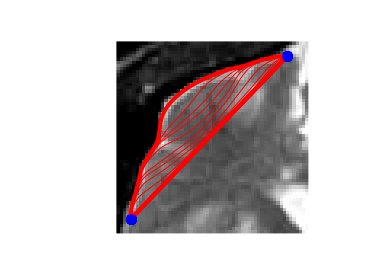# Active Contours using Parameteric Curves¶

This tour explores image segmentation using parametric active contours. $\newcommand{\dotp}{\langle #1, #2 \rangle}$ $\newcommand{\qandq}{\quad\text{and}\quad}$ $\newcommand{\qwhereq}{\quad\text{where}\quad}$ $\newcommand{\qifq}{ \quad \text{if} \quad }$ $\newcommand{\ZZ}{\mathbb{Z}}$ $\newcommand{\RR}{\mathbb{R}}$ $\newcommand{\CC}{\mathbb{C}}$ $\newcommand{\pa}{\left(#1\right)}$ $\newcommand{\si}{\sigma}$ $\newcommand{\Nn}{\mathcal{N}}$ $\newcommand{\Bb}{\mathcal{B}}$ $\newcommand{\EE}{\mathbb{E}}$ $\newcommand{\norm}{\|#1\|}$ $\newcommand{\abs}{\left|#1\right|}$ $\newcommand{\choice}{ \left\{ \begin{array}{l} #1 \end{array} \right. }$ $\newcommand{\al}{\alpha}$ $\newcommand{\la}{\lambda}$ $\newcommand{\ga}{\gamma}$ $\newcommand{\Ga}{\Gamma}$ $\newcommand{\La}{\Lambda}$ $\newcommand{\si}{\sigma}$ $\newcommand{\Si}{\Sigma}$ $\newcommand{\be}{\beta}$ $\newcommand{\de}{\delta}$ $\newcommand{\De}{\Delta}$ $\newcommand{\phi}{\varphi}$ $\newcommand{\th}{\theta}$ $\newcommand{\om}{\omega}$ $\newcommand{\Om}{\Omega}$

In :
from __future__ import division
from nt_toolbox.general import *
from nt_toolbox.signal import *
%pylab inline
%matplotlib inline

Populating the interactive namespace from numpy and matplotlib

WARNING: pylab import has clobbered these variables: ['pylab']
%matplotlib prevents importing * from pylab and numpy


## Parameteric Curves¶

In this tours, the active contours are represented using parametric curve $\ga : [0,1] \rightarrow \RR^2$.

This curve is discretized using a piewise linear curve with $p$ segments, and is stored as a complex vector of points in the plane $\ga \in \CC^p$.

Initial polygon.

In :
gamma0 = array([.78, .14, .42, .18, .32, .16, .75, .83, .57, .68, .46, .40, .72, .79, .91, .90]) + 1j*array([.87, .82, .75, .63, .34, .17, .08, .46, .50, .25, .27, .57, .73, .57, .75, .79])


Display the initial curve.

In :
periodize = lambda gamma: concatenate((gamma, [gamma]))
def cplot(gamma,s='b',lw=1):
plot(real(periodize(gamma)), imag(periodize(gamma)), s, linewidth=lw)
axis('equal')
axis('off')
cplot(gamma0,'b.-');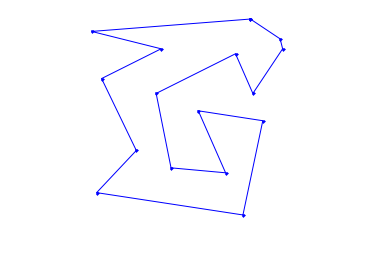Number of points of the discrete curve.

In :
p = 256


Shortcut to re-sample a curve according to arc length.

In :
interpc = lambda x,xf,yf: interp(x,xf,real(yf)) + 1j * interp(x,xf,imag(yf))
curvabs = lambda gamma: concatenate( (, cumsum( 1e-5 + abs(gamma[:-1:]-gamma[1::]) ) ) )
resample1 = lambda gamma,d: interpc(arange(0,p)/float(p),  d/d[-1],gamma)
resample = lambda gamma: resample1( periodize(gamma), curvabs(periodize(gamma)) )


Initial curve $\ga_1(t)$.

In :
gamma1 = resample(gamma0)


Display the initial curve.

In :
cplot(gamma1, 'k')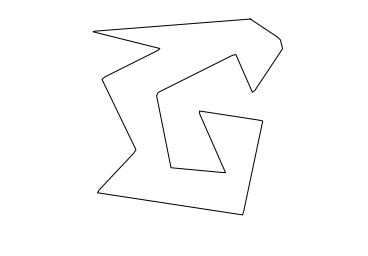Shortcut for forward and backward finite differences.

In :
shiftR = lambda c: concatenate( ([c[-1]],c[:-1:]) )
shiftL = lambda c: concatenate( (c[1::],[c]) )
BwdDiff = lambda c: c - shiftR(c)
FwdDiff = lambda c: shiftL(c) - c


The tangent to the curve is computed as $$t_\ga(s) = \frac{\ga'(t)}{\norm{\ga'(t)}}$$ and the normal is $n_\ga(t) = t_\ga(t)^\bot.$

Shortcut to compute the tangent and the normal to a curve.

In :
normalize = lambda v: v/maximum(abs(v),1e-10)
tangent = lambda gamma: normalize( FwdDiff(gamma) )
normal = lambda gamma: -1j*tangent(gamma)


Move the curve in the normal direction, by computing $\ga_1(t) \pm \delta n_{\ga_1}(t)$.

In :
delta = .03
gamma2 = gamma1 + delta * normal(gamma1)
gamma3 = gamma1 - delta * normal(gamma1)


Display the curves.

In :
cplot(gamma1, 'k')
cplot(gamma2, 'r--')
cplot(gamma3, 'b--')
axis('tight')
axis('off')

Out:
(0.13822079913787064,
0.93899831377290144,
0.053329237592183561,
0.89990886424047734)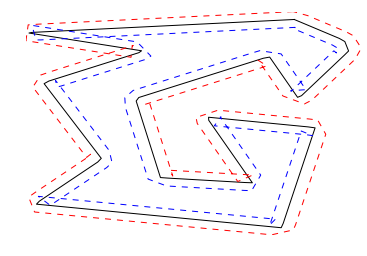## Evolution by Mean Curvature¶

A curve evolution is a series of curves $s \mapsto \ga_s$ indexed by an evolution parameter $s \geq 0$. The intial curve $\ga_0$ for $s=0$ is evolved, usually by minizing some energy $E(\ga)$ in a gradient descent $$\frac{\partial \ga_s}{\partial s} = \nabla E(\ga_s).$$

Note that the gradient of an energy is defined with respect to the curve-dependent inner product $$\dotp{a}{b} = \int_0^1 \dotp{a(t)}{b(t)} \norm{\ga'(t)} d t.$$ The set of curves can thus be thought as being a Riemannian surface.

The simplest evolution is the mean curvature evolution. It corresponds to minimization of the curve length $$E(\ga) = \int_0^1 \norm{\ga'(t)} d t$$

The gradient of the length is $$\nabla E(\ga)(t) = -\kappa_\ga(t) n_\ga(t)$$ where $\kappa_\ga$ is the curvature, defined as $$\kappa_\ga(t) = \frac{1}{\norm{\ga'(t)}} \dotp{ t_\ga'(t) }{ n_\ga(t) } .$$

Shortcut for normal times curvature $\kappa_\ga(t) n_\ga(t)$.

In :
normalC = lambda gamma: BwdDiff(tangent(gamma)) / abs( FwdDiff(gamma) )


Time step for the evolution. It should be very small because we use an explicit time stepping and the curve has strong curvature.

In :
dt = 0.001 / 100


Number of iterations.

In :
Tmax = 3.0 / 100
niter = round(Tmax/dt)


Initialize the curve for $s=0$.

In :
gamma = gamma1


Evolution of the curve.

In :
gamma = gamma + dt * normalC(gamma)


To stabilize the evolution, it is important to re-sample the curve so that it is unit-speed parametrized. You do not need to do it every time step though (to speed up).

In :
gamma = resample(gamma)


Exercise 1: Perform the curve evolution. You need to resample it a few times.

In :
run -i nt_solutions/segmentation_2_snakes_param/exo1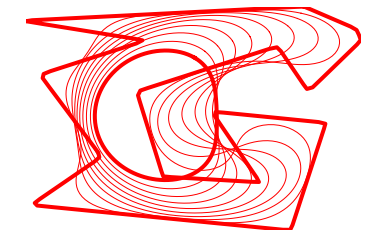## Geodesic Active Contours¶

Geodesic active contours minimize a weighted length $$E(\ga) = \int_0^1 W(\ga(t)) \norm{\ga'(t)} d t,$$ where $W(x)>0$ is the geodesic metric, that should be small in areas where the image should be segmented.

Size of the image $n$.

In :
n = 200


Create a synthetic weight $W(x)$.

In :
nbumps = 40
theta = random.rand(nbumps,1)*2*pi
r = .6*n/2
a = array([.62*n,.6*n])
x = around( a + r*cos(theta) )
y = around( a + r*sin(theta) )
W = zeros([n,n])
for i in arange(0,nbumps):
W[int(x[i]),int(y[i])] = 1
W = gaussian_blur(W,6.0)
W = rescale( -minimum(W,.05), .3,1)


Display the metric $W$.

In :
imageplot(W)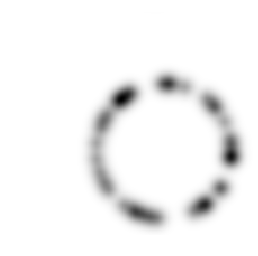Pre-compute the gradient $\nabla W(x)$ of the metric.

In :
G = grad(W)
G = G[:,:,0] + 1j*G[:,:,1]


Display the image of the magnitude $\norm{\nabla W(x)}$ of the gradient.

In :
imageplot(abs(G))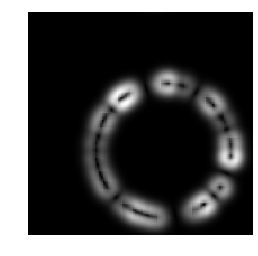Shortcut to evaluate the gradient and the potential along a curve.

In :
EvalG = lambda gamma: bilinear_interpolate(G, imag(gamma), real(gamma))
EvalW = lambda gamma: bilinear_interpolate(W, imag(gamma), real(gamma))


Create a circular curve $\ga_0$.

In :
r = .98*n/2 # radius
p = 128 # number of points on the curve
theta = transpose( linspace(0, 2*pi, p + 1) )
theta = theta[0:-1]
gamma0 = n/2 * (1 + 1j) +  r*(cos(theta) + 1j*sin(theta))


Initialize the curve at time $t=0$ with a circle.

In :
gamma = gamma0


For this experiment, the time step should be larger, because the curve is in $[0,n-1] \times [0,n-1]$.

In :
dt = 1


Number of iterations.

In :
Tmax = 5000
niter = round(Tmax/ dt)


Display the curve on the background.

In :
lw = 2
clf
imageplot(transpose(W))
cplot(gamma, 'r', lw)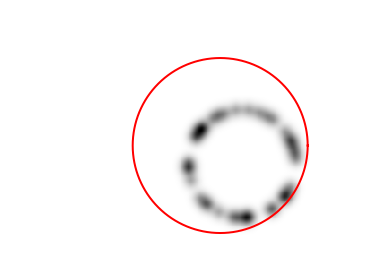The gradient of the energy is $$\nabla E(\ga) = -W(\ga(t)) \kappa_\ga(t) n_\ga(t) + \dotp{\nabla W(\ga(t))}{ n_\ga(t) } n_\ga(t).$$

Pointwise innerproduct on the curve.

In :
dotp = lambda c1,c2: real(c1)*real(c2) + imag(c1)*imag(c2)


Evolution of the curve according to this gradient.

In :
N = normal(gamma)
g = - EvalW(gamma) * normalC(gamma) + dotp(EvalG(gamma), N) * N
gamma = gamma - dt*g


To avoid the curve from being poorly sampled, it is important to re-sample it evenly.

In :
gamma = resample(gamma)


Exercise 2: Perform the curve evolution.

In :
run -i nt_solutions/segmentation_2_snakes_param/exo2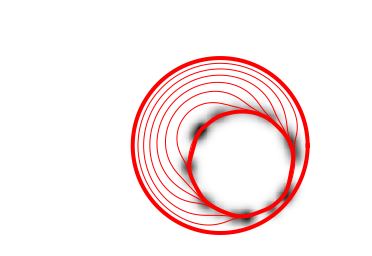# Medical Image Segmentation¶

One can use a gradient-based metric to perform edge detection in medical images.

Load an image $f$.

In :
n = 256
name = 'nt_toolbox/data/cortex.bmp'


Display.

In :
imageplot(f)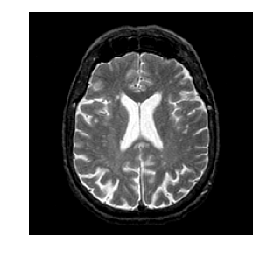An edge detector metric can be defined as a decreasing function of the gradient magnitude. $$W(x) = \psi( d \star h_a(x) ) \qwhereq d(x) = \norm{\nabla f(x)}.$$ where $h_a$ is a blurring kernel of width $a>0$.

Compute the magnitude of the gradient.

In :
G = grad(f)
d0 = sqrt(sum(G**2, 2))
imageplot(d0)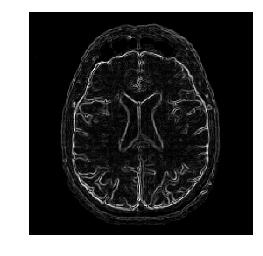Blur it by $h_a$.

In :
a = 2
d = gaussian_blur(d0, a)
imageplot(d)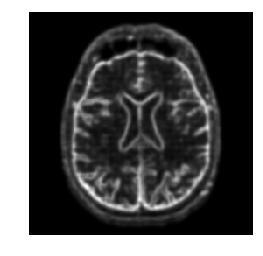Compute a decreasing function of the gradient to define $W$.

In :
d = minimum(d, .4)
W = rescale(-d, .8, 1)


Display it.

In :
imageplot(W)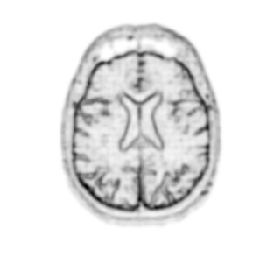Number of points.

In :
p = 128


Exercise 3: Create an initial circle $\gamma_0$ of $p$ points. When plotting the image, you need to transpose it to have axis coherent with the cplot.

In :
run -i nt_solutions/segmentation_2_snakes_param/exo3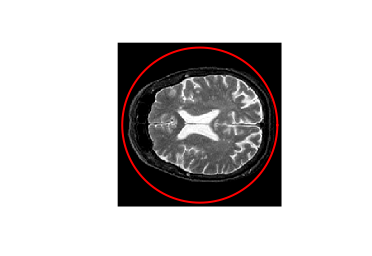Step size.

In :
dt = 2


Number of iterations.

In :
Tmax = 9000
niter = round(Tmax/ dt)


Exercise 4: Perform the curve evolution.

In :
run -i nt_solutions/segmentation_2_snakes_param/exo4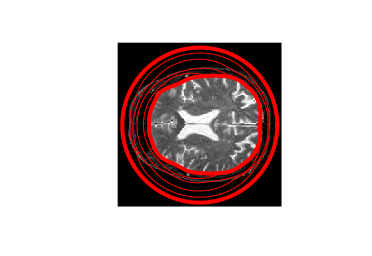# Evolution of a Non-closed Curve¶

It is possible to perform the evolution of a non-closed curve by adding boundary constraint $$\ga(0)=x_0 \qandq \ga(1)=x_1.$$

In this case, the algorithm find a local minimizer of the geodesic distance between the two points.

Note that a much more efficient way to solve this problem is to use the Fast Marching algorithm to find the global minimizer of the geodesic length.

Load an image $f$.

In :
n = 256
f = f[45:105, 60:120]
n = f.shape


Display.

In :
imageplot(f)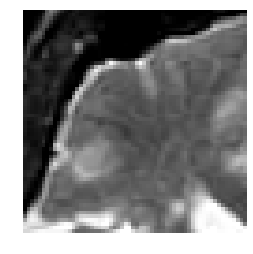Exercise 5: Compute an edge attracting criterion $W(x)>0$, that is small in area of strong gradient.

In :
run -i nt_solutions/segmentation_2_snakes_param/exo5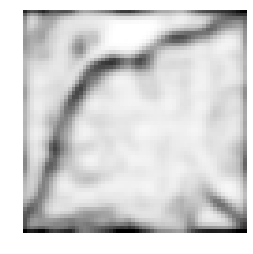Start and end points $x_0$ and $x_1$.

In :
x0 = 4 + 55j
x1 = 53 + 4j


Initial curve $\ga_0$.

In :
p = 128
t = transpose(linspace(0, 1, p))
gamma0 = t*x1 + (1-t)*x0


Initialize the evolution.

In :
gamma = gamma0


Display.

In :
clf
imageplot(transpose(W))
cplot(gamma,'r', 2)
plot(real(gamma), imag(gamma), 'b.', markersize=20)
plot(real(gamma[-1]), imag(gamma[-1]), 'b.', markersize=20);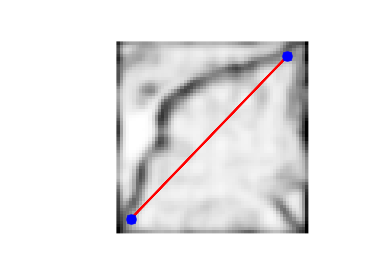Re-sampling for non-periodic curves.

In :
curvabs = lambda gamma: concatenate( (, cumsum( 1e-5 + abs(gamma[:-1:]-gamma[1::]) ) ) )
resample1 = lambda gamma,d: interpc(arange(0,p)/float(p-1),  d/d[-1],gamma)
resample = lambda gamma: resample1( gamma, curvabs(gamma) )


Time step.

In :
dt = 1/10


Number of iterations.

In :
Tmax = 2000*4/ 7
niter = round(Tmax/ dt)


Exercise 6: Perform the curve evolution. Be careful to impose the boundary conditions at each step.

In :
run -i nt_solutions/segmentation_2_snakes_param/exo6